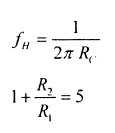Courses

# Analog Electronics - MCQ Test - 2

## 20 Questions MCQ Test GATE ECE (Electronics) 2022 Mock Test Series | Analog Electronics - MCQ Test - 2

Description
This mock test of Analog Electronics - MCQ Test - 2 for Electronics and Communication Engineering (ECE) helps you for every Electronics and Communication Engineering (ECE) entrance exam. This contains 20 Multiple Choice Questions for Electronics and Communication Engineering (ECE) Analog Electronics - MCQ Test - 2 (mcq) to study with solutions a complete question bank. The solved questions answers in this Analog Electronics - MCQ Test - 2 quiz give you a good mix of easy questions and tough questions. Electronics and Communication Engineering (ECE) students definitely take this Analog Electronics - MCQ Test - 2 exercise for a better result in the exam. You can find other Analog Electronics - MCQ Test - 2 extra questions, long questions & short questions for Electronics and Communication Engineering (ECE) on EduRev as well by searching above.
QUESTION: 1

### Shown below is a timer 555 IC circuit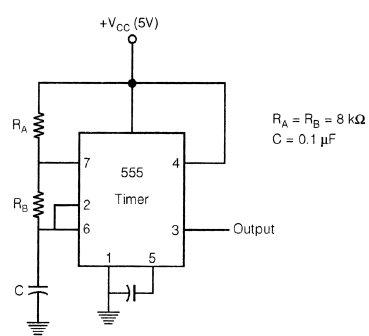Frequency of the waveform generated by the above circuit is

Solution: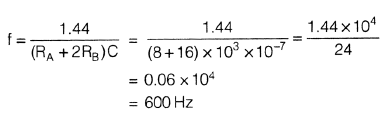QUESTION: 2

### The circuit shown in figure below represents: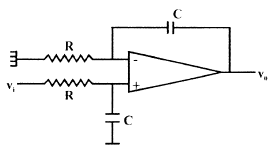Solution:

It is just by figure only. But one can also calculate transfer function.

*Answer can only contain numeric values
QUESTION: 3

### Consider the following feedback pair connection.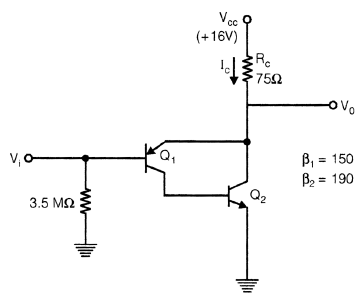Collector current IC is ____________ mA.

Solution: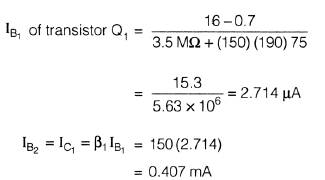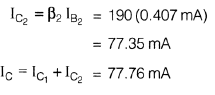QUESTION: 4

If for a given programmable OPAMP, G1 is the gain, when only S1 is closed and G2 is the gain when only S2 is closed, then relation between G1 & G2 is?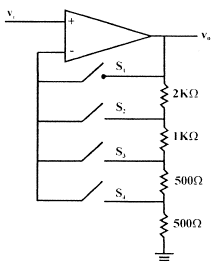Solution:

When S1 is closed G1 = 1 it behaves like a voltage follower circuit. When S2 s closed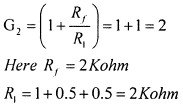QUESTION: 5

For given RC − Phase shift oscillator, by use of BJT if R1 = R2 = 10k, Rc = 2K, R = 2K, C = 0.01 µF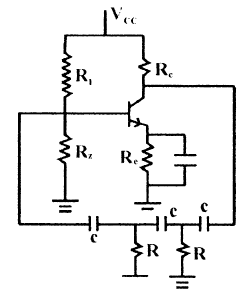Q.

What is Minimum value of hfe for possible oscillation?

Solution: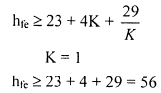QUESTION: 6

For given RC − Phase shift oscillator, by use of BJT if R1 = R2 = 10k, Rc = 2K, R = 2K, C = 0.01 µFQ.

What is frequency of oscillation for circuit?

Solution: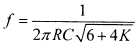QUESTION: 7

For a given CE, amplifier if β = 100, IC = 1 mA, RS = 1 KΩ, Rc = 5 KΩ, Re = 200 Ω, hie = 2.5 K.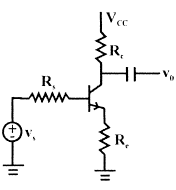Q.

Calculate value of voltage gain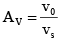Solution: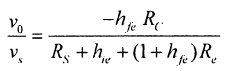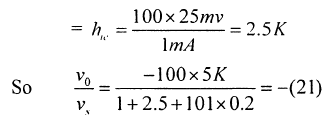QUESTION: 8

For a given CE, amplifier if β = 100, IC = 1 mA, RS = 1 KΩ, Rc = 5 KΩ, Re = 200 Ω, hie = 2.5 K.Q.

Calculate input impedance seen by source

Solution: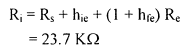QUESTION: 9

For the given FET, circuit if

RD = 20 kΩ rd = 50 kΩ
RS = 2 kΩ µ = 50Q.

Calculate value of v0/vs

Solution: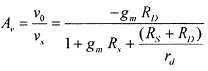QUESTION: 10

For the given FET, circuit if

RD = 20 kΩ rd = 50 kΩ
RS = 2 kΩ µ = 50Q.

Calculate value of output impedance

Solution:QUESTION: 11

The output voltage V0 for given circuit is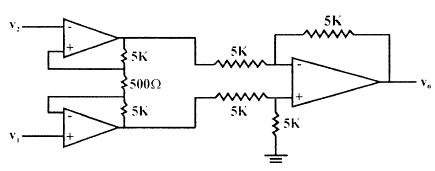Solution:*Answer can only contain numeric values
QUESTION: 12

A MOSFET amplifier circuit is as shown below:

If device parameter K = 15 µA/V2, then value of Transconductance of MOSFET is _________ ms.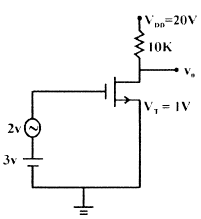Solution: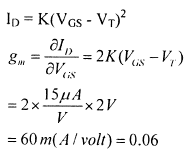QUESTION: 13

The 3-terminal linear voltage regulator is connected to a 10 ohm load resistor as shown in figure. If Vin is 10 volt, what is power dissipated in the Si transistor.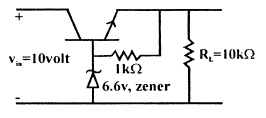Solution:
QUESTION: 14

A sinusoidal oscillator is built using an amplifier of real gain A, infinite input impedance and feedback (β) network is shown in figure. If Z = jX, then what is the value of z0.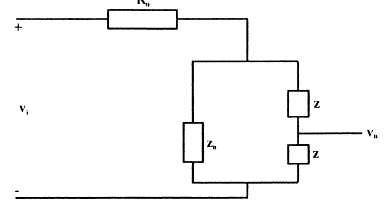Solution:

Z+Z+Z0 =0 ⇒ Z0 = - 2jX

QUESTION: 15

Shown below is a network, in collector feedback mode.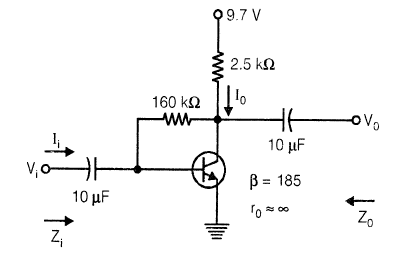Q.

The voltage gain Av is ……………., Assume re =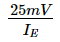Solution: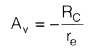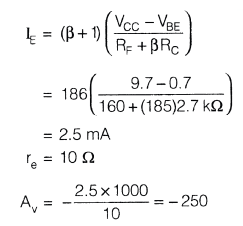QUESTION: 16

Shown below is a network, in collector feedback mode.Q.

The current gain Ai is ≈

Solution: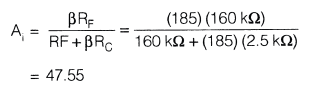QUESTION: 17

A power amplifier delivers 250 watt output at 50% efficiency. If ambient temp. is 25°C and maxm allowable junction temp. is 150°C, then what is value of Maximum thermal resistance which can be tolerated.

Solution: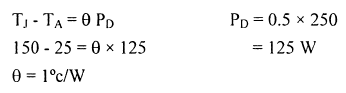*Answer can only contain numeric values
QUESTION: 18

Numerical Anser type question

Q.

For a BJT, CE-mode, Base to emitter capacitance (Cπ) is 10 times the collector to base capacitance (Cµ). If transistor is biased at quiescent collector current ICQ = 1 mA, and its short circuit unity gain frequency is 0.909 M rad/sec, then the value of Cπ is _______ nF.

Solution: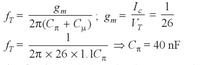QUESTION: 19

In voltage reference diodes:

Solution:

Voltage reference diode means zener diode

QUESTION: 20

What should be values of components R & R2 such that frequency of wein-bridge oscillator is 300 HZ, if C = 0.01 µF, R1 = 12 kΩ.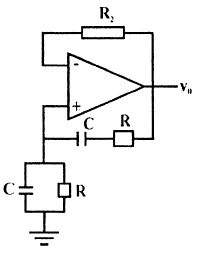Solution: